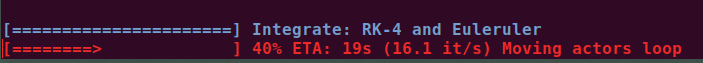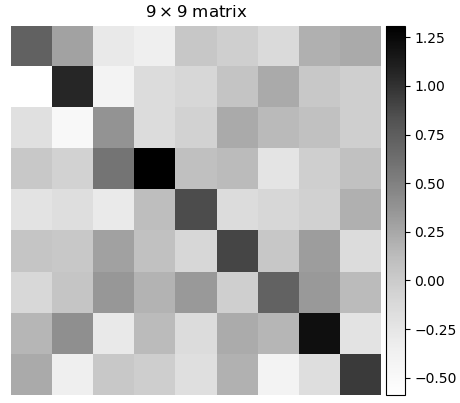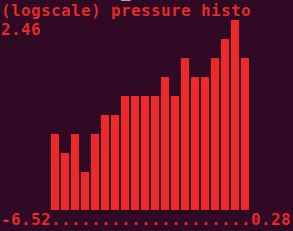# utils¶

Utilities submodule.

## ProgressBar¶

class vtkplotter.utils.ProgressBar(start, stop, step=1, c=None, ETA=True, width=24, char='▬')[source]

Bases: object

Class to print a progress bar with optional text message.

Example: import time pb = ProgressBar(0,400, c='red') for i in pb.range(): time.sleep(.1) pb.print('some message') # or pb.print(counts=i)len()[source]

Return the number of steps.

print(txt='', counts=None)[source]

Print the progress bar and optional message.

range()[source]

Return the range iterator.

## cameraFromNeuroglancer¶

vtkplotter.utils.cameraFromNeuroglancer(state, zoom=300)[source]

Define a vtkCamera from a neuroglancer state dictionary.

Parameters: state (dict) – an neuroglancer state dictionary. zoom (float) – how much to multiply zoom by to get camera backoff distance default = 300 > ngl_zoom = 1 > 300 nm backoff distance. a vtk camera setup that matches this state. vtk.vtkCamera

## cameraFromQuaternion¶

vtkplotter.utils.cameraFromQuaternion(pos, quaternion, distance=10000, ngl_correct=True)[source]

Define a vtkCamera with a particular orientation.

Parameters: pos (np.array, list, tuple) – an iterator of length 3 containing the focus point of the camera quaternion (np.array, list, tuple) – a len(4) quaternion (x,y,z,w) describing the rotation of the camera such as returned by neuroglancer x,y,z,w all in [0,1] range distance (float) – the desired distance from pos to the camera (default = 10000 nm) a vtk camera setup according to these rules. vtk.vtkCamera

## cart2pol¶

vtkplotter.utils.cart2pol(x, y)[source]

Cartesian to Polar coordinates conversion.

## cart2spher¶

vtkplotter.utils.cart2spher(x, y, z)[source]

Cartesian to Spherical coordinate conversion.

## fitCircle2D¶

vtkplotter.utils.fitCircle2D(points)[source]

Fits a circle through points in the plane, with a very fast non-iterative method.

Returns the tuple (center_x, center_y, radius).

Reference: J.F. Crawford, Nucl. Instr. Meth. 211, 1983, 223-225.

Note

E.g.:

from vtkplotter import *

x = arange(0, 3, 0.1)
y = sin(x)

cx, cy, R = fitCircle2D([x,y])
Points([x, y])
Point([cx, cy])
Circle([cx, cy], r=R)
show(..., axes=8)


## geometry¶

vtkplotter.utils.geometry(obj, extent=None)[source]

Apply the vtkGeometryFilter. This is a general-purpose filter to extract geometry (and associated data) from any type of dataset. This filter also may be used to convert any type of data to polygonal type. The conversion process may be less than satisfactory for some 3D datasets. For example, this filter will extract the outer surface of a volume or structured grid dataset.

Returns an Actor object.

Parameters: extent (list) – set a [xmin,xmax, ymin,ymax, zmin,zmax] bounding box to clip data.

## grep¶

vtkplotter.utils.grep(filename, tag, firstOccurrence=False)[source]

Greps the line that starts with a specific tag string from inside a file.

## humansort¶

vtkplotter.utils.humansort(l)[source]

Sort in place a given list the way humans expect.

NB: input list is modified

E.g. [‘file11’, ‘file1’] -> [‘file1’, ‘file11’]

## isSequence¶

vtkplotter.utils.isSequence(arg)[source]

Check if input is iterable.

## linInterpolate¶

vtkplotter.utils.linInterpolate(x, rangeX, rangeY)[source]

Interpolate linearly variable x in rangeX onto rangeY. If x is a vector the linear weight is the distance to two the rangeX vectors.

E.g. if x runs in rangeX=[x0,x1] and I want it to run in rangeY=[y0,y1] then y = linInterpolate(x, rangeX, rangeY) will interpolate x onto rangeY.

## mag¶

vtkplotter.utils.mag(z)[source]

Get the magnitude of a vector.

## mag2¶

vtkplotter.utils.mag2(z)[source]

Get the squared magnitude of a vector.

## makeBands¶

vtkplotter.utils.makeBands(inputlist, numberOfBands)[source]

Group values of a list into bands of equal value.

Parameters: numberOfBands (int) – number of bands, a positive integer > 2. a binned list of the same length as the input.

## orientedCamera¶

vtkplotter.utils.orientedCamera(center=(0, 0, 0), upVector=(0, 1, 0), backoffVector=(0, 0, 1), backoff=1)[source]

Generate a vtkCamera pointed at a specific location, oriented with a given up direction, set to a backoff.

## plotMatrix¶

vtkplotter.utils.plotMatrix(M, title='matrix', continuous=True, cmap='Greys')[source]
Plot a matrix using matplotlib.
Example: from vtkplotter.dolfin import plotMatrix import numpy as np M = np.eye(9) + np.random.randn(9,9)/4 plotMatrix(M)## pointIsInTriangle¶

vtkplotter.utils.pointIsInTriangle(p, p1, p2, p3)[source]

Return True if a point is inside (or above/below) a triangle defined by 3 points in space.

## pointToLineDistance¶

vtkplotter.utils.pointToLineDistance(p, p1, p2)[source]

Compute the distance of a point to a line (not the segment) defined by p1 and p2.

## pol2cart¶

vtkplotter.utils.pol2cart(theta, rho)[source]

Polar to Cartesian coordinates conversion.

## precision¶

vtkplotter.utils.precision(x, p, vrange=None)[source]

Returns a string representation of x formatted with precision p.

Parameters: vrange (float) – range in which x exists (to snap it to ‘0’ if below precision).

Based on the webkit javascript implementation taken from here, and implemented by randlet.

## printHistogram¶

vtkplotter.utils.printHistogram(data, bins=10, height=10, logscale=False, minbin=0, horizontal=False, char='▉', c=None, bold=True, title='Histogram')[source]

Ascii histogram printing. Input can also be Volume or Actor. Returns the raw data before binning (useful when passing vtk objects).

Parameters: bins (int) – number of histogram bins height (int) – height of the histogram in character units logscale (bool) – use logscale for frequencies minbin (int) – ignore bins before minbin horizontal (bool) – show histogram horizontally char (bool) – character to be used c (str,int) – ascii color char – use boldface title (str) – histogram title from vtkplotter import printHistogram import np as np d = np.random.normal(size=1000) data = printHistogram(d, c='blue', logscale=True, title='my scalars') data = printHistogram(d, c=1, horizontal=1) print(np.mean(data)) # data here is same as d## printInfo¶

vtkplotter.utils.printInfo(obj)[source]

Print information about a vtk object.

## resampleArrays¶

vtkplotter.utils.resampleArrays(source, target, tol=None)[source]

Resample point and cell data of a dataset on points from another dataset.

Parameters: tol (float) – set the tolerance used to compute whether a point in the target is in a cell of the source. Points without resampled values, and their cells, are be marked as blank.

## spher2cart¶

vtkplotter.utils.spher2cart(rho, theta, phi)[source]

Spherical to Cartesian coordinate conversion.

## trimesh2vtk¶

vtkplotter.utils.trimesh2vtk(inputobj, alphaPerCell=False)[source]

Convert Trimesh object to Actor(vtkActor) or Assembly object.

## vector¶

vtkplotter.utils.vector(x, y=None, z=0.0, dtype=<class 'numpy.float64'>)[source]

Return a 3D numpy array representing a vector.

If y is None, assume input is in the form [x,y,z].

## versor¶

vtkplotter.utils.versor(v)[source]

Return the unit vector. Input can be a list of vectors.

## vtk2trimesh¶

vtkplotter.utils.vtk2trimesh(actor)[source]

Convert vtk Actor to Trimesh object.

## vtkCameraToK3D¶

vtkplotter.utils.vtkCameraToK3D(vtkcam)[source]

Convert a vtkCamera object into a 9-element list to be used by K3D backend.

Output format is: [posx,posy,posz, targetx,targety,targetz, upx,upy,upz]